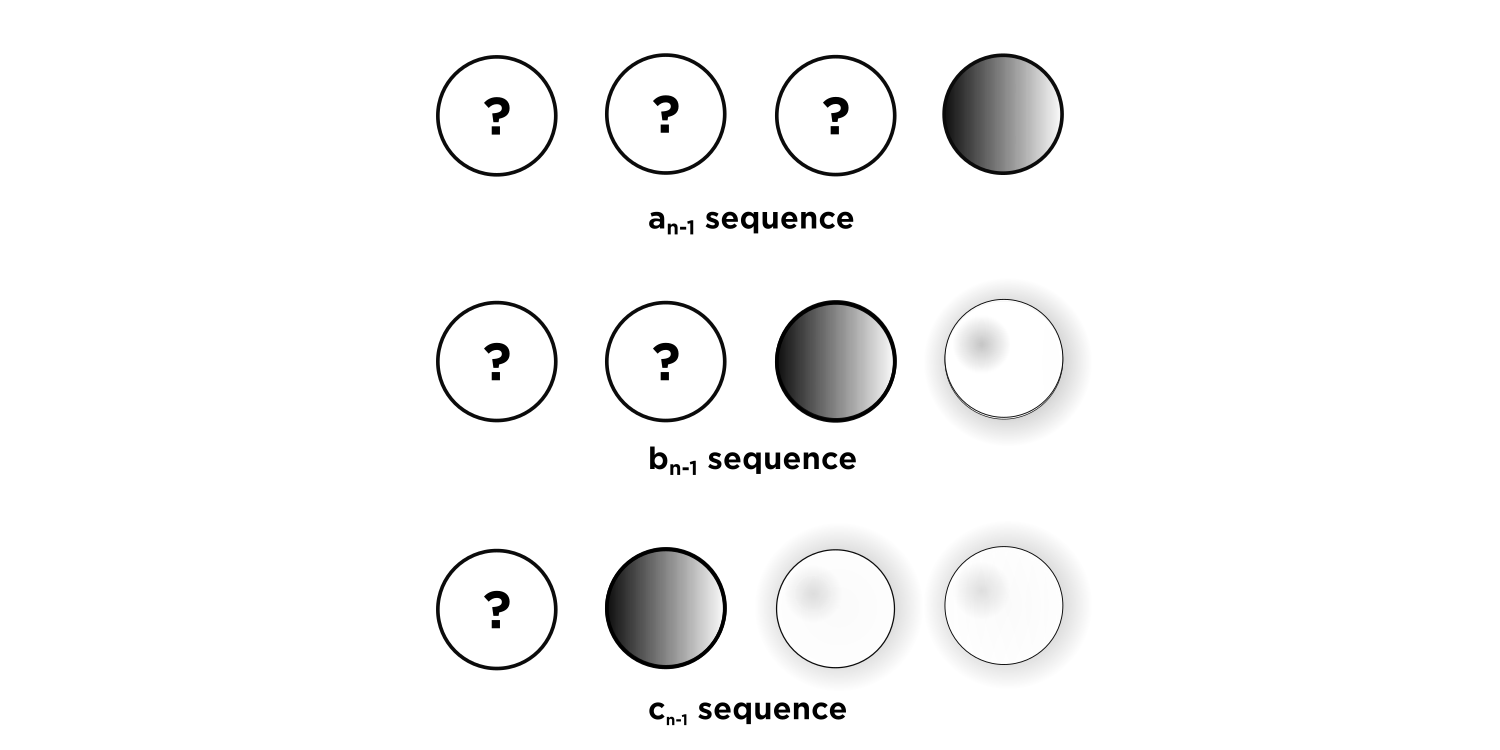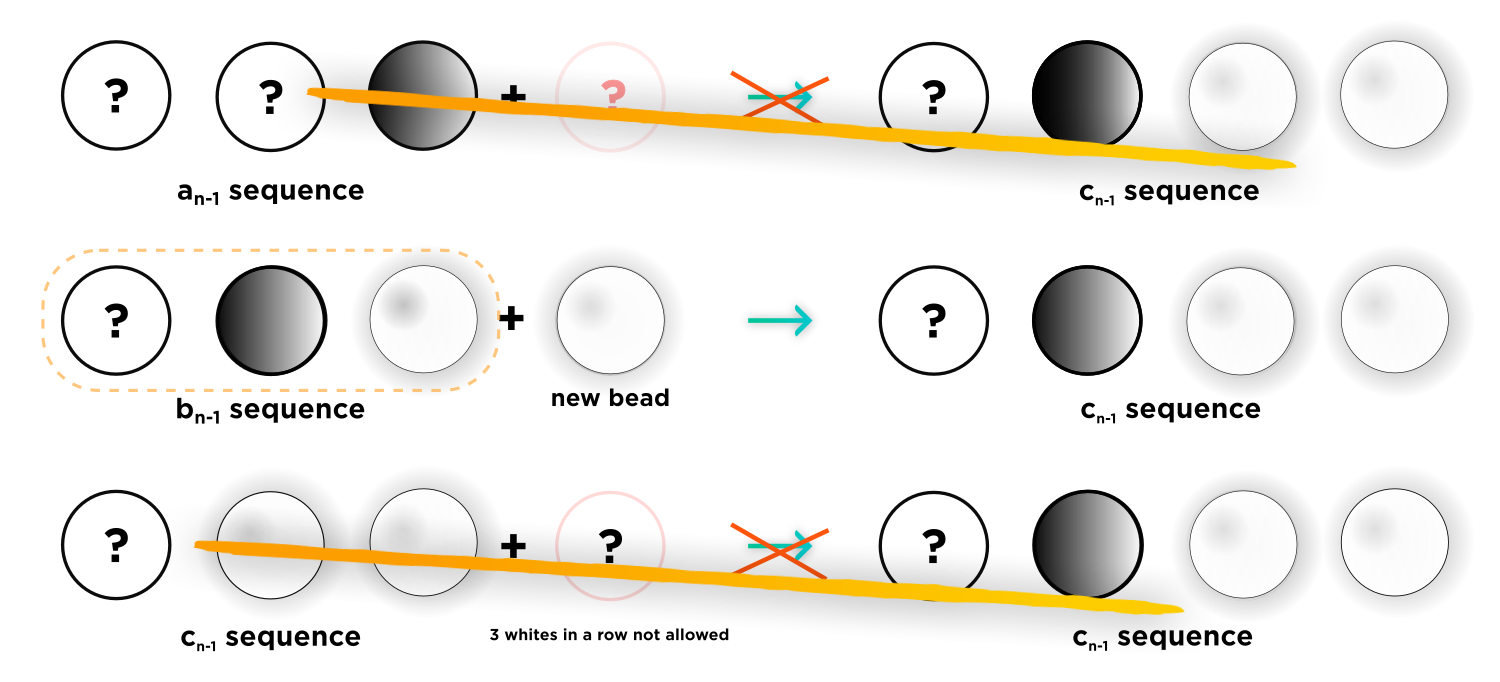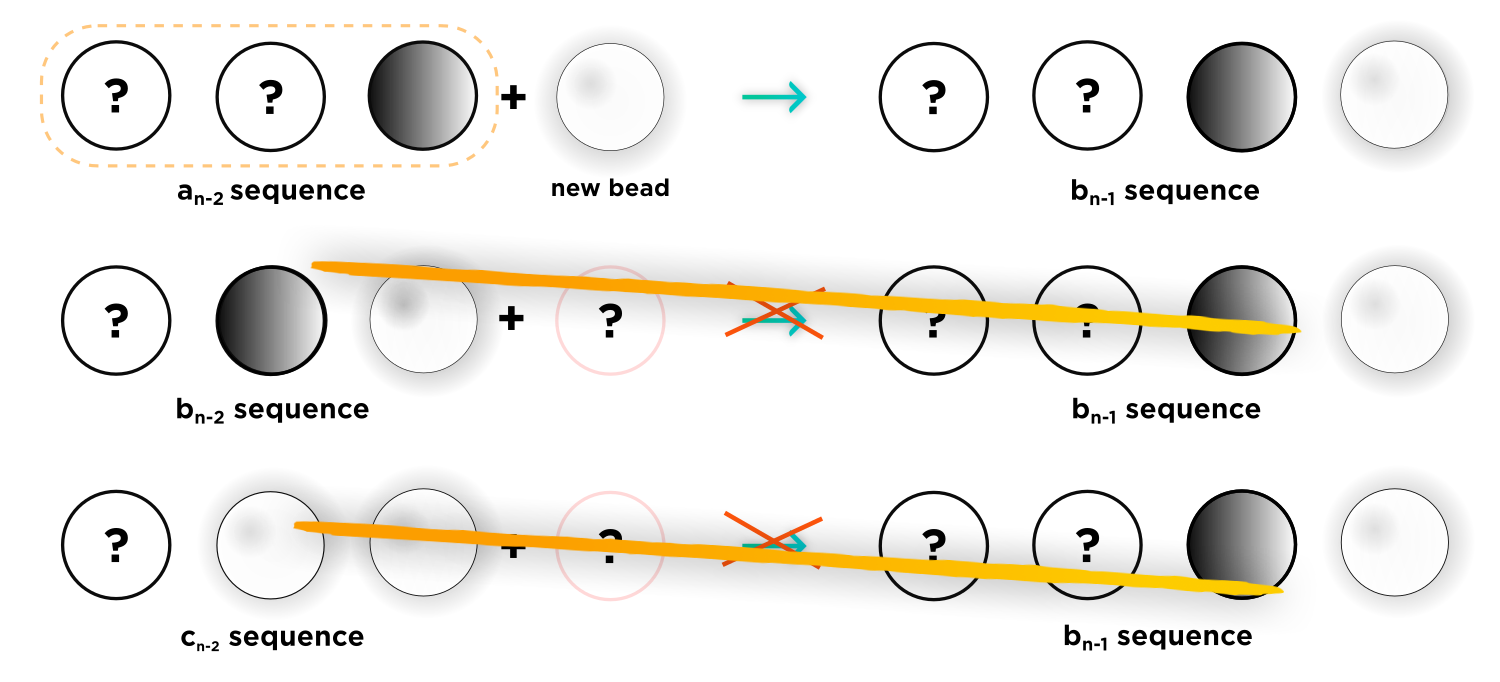# Can you explain why the answer isn't aₙ₋₂ ​ ?

• Module 3 Week 3 Day 11 Your Turn Part 1 Mini-Question

I don't understand the answer explanation. Also, can you explain why the answer isn't $$a_{n-2}$$? If there are two white beads at the end, and you can't have any more whites next to it, then you have to something ending in black, aka $$a_n$$. And if you want the length to be correct, it has to be $$a_{n-2}$$ (since there are already two white beads).

• @divinedolphin This is such a clever comment! I really like it! You are absolutely correct that another possible answer is

$$c_n = a_{n-2}$$

It just doesn't happen to be one of the answer choices.Let's take a look at the given solution provided first. Our sequences are: $$a_{n},$$ denoting sequences ending with a black bead, $$b_n,$$ denoting sequences ending with only one white bead, and $$c_n,$$ denoting sequences ending with two white beads.In order to make a $$c_n$$ sequence ending with two white beads, we can start with a $$b_{n-1}$$ sequence of length $$n-1$$ ending with one white bead. We cannot start with an $$a_{n-1}$$ sequence, since that would give us $$\textcolor{blue}{{BW}},$$ and we cannot start with a $$c_{n-1}$$ sequence, since that would give us $$\textcolor{red}{WWW},$$ which isn't allowed.The number of $$\textcolor{blue}{WW}$$ sequences is exactly the number of $$\textcolor{blue}{BW}$$ sequences of length $$n-1.$$

$$\boxed{ c_n = b_{n-1}}$$

This was the given answer to the mini-question.

Now we can iterate one step farther back. Let's think; how do we get a $$b_{n-1}$$ sequence?If we start with a $$b_{n-2}$$ sequence and add a white bead, we'll get two white beads at the end, which isn't a $$b_{n-1}$$ sequence. If we start with a $$c_{n-2}$$ sequence and add a white bead, we'll get three beads at the end, which isn't allowed. The only way to get a $$b_{n-1}$$ sequence is to start with a $$a_{n-2}$$ sequence, which ends in black, and to add a white bead at the end.

$$b_{n-1} = a_{n-2}$$

And from before, we know

\begin{aligned} c_{n} &= b_{n-1} \\ &= a_{n-1} \\ \end{aligned}

So you are correct in thinking that $$a_{n-2}$$ could be another possible answer choice.• @debbie
Maybe this should be a multi-answer question?

• @rz923 Thank you for supporting this suggestion! This would be a really clever addition to the problem. I will note it down.